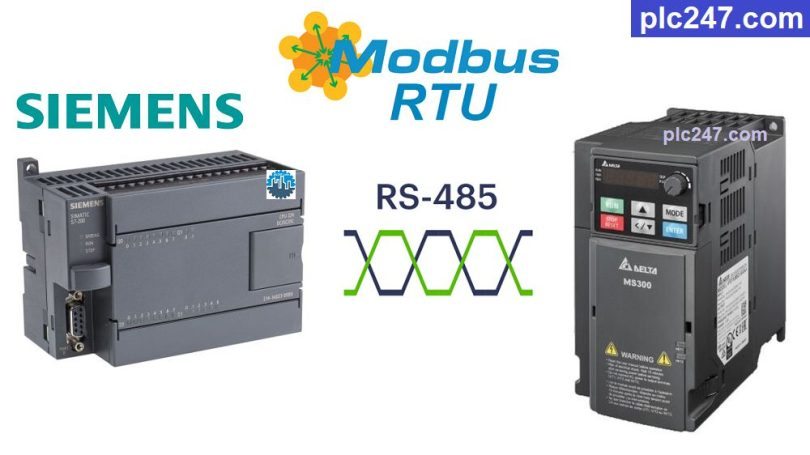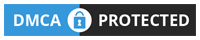# Siemens S7-200 “Modbus RTU” Delta MS300 VFDWritten by

M300 series inverter is a new generation inverter from Delta, this is considered Delta’s most beautiful inverter line today. Extremely compact design, high aesthetics, outstanding performance and powerful configuration meet a variety of applications for industrial automation machines.

In addition to the basic connections required of the inverter, Delta MS300 also supports Modbus-RTU communication protocol, today plc247.com will guide you to control it with Siemens S7-200 PLC through this protocol.

#### Siemens S7-200 & Delta M300 “Connection Diagram”

Below is the RS485 communication connection diagram between PLC Siemens S7-200 and Delta MS300 inverter.

#### Delta MS300 Modbus Parameters Setting

Below are the communication parameters that need to be installed for Delta MS300 inverter to make it controllable and monitored using Modbus RTU protocol

+ P00.20 = 1 (Using Modbus Setting Frequency)

+ P00.21 = 2 (Using Modbus Control Motor)

+ P09.00 = 1 (Slave Address = 1)

+ P09.01 = 9.6 (Baudrate = 9600bps)

+ P09.02 = 3

+ P09.03 = 1.0 (Overtime Com. = 1.0s)

+ P09.04 = 14 ( Modbus-RTU 8/E/1 Selection)

##### Note: Siemens S7-200 Holding Registers Address = “VFD Address (Dec) + 40001“

>>> Motor Control Address = 2000 (hex) + 40001
= 8192 (dec) + 40001 = 48193 (dec)

• Set Word 48193 = 18 (dec): Forward Run
• Set Word 48193 = 34 (dec): Reverse Run
• Set Word 48193 = 1 (dec): Stop

>>> Frequency Setting Address = 2001 (hex) + 40001
= 8193 (dec) + 40001 = 48194 (dec)

+ Output Frequency Address = 48452 (dec)

+ Output Current Address = 48453 (dec)

+ Output Volatge Address = 48455 (dec)

### PLC Programming

+ Modbus Configuration

+ Frequency Write Command

+ VFD Control Command

### Project Video Tutorial

======

###### Related Software and Documents